# SAT Math Multiple Choice Question 895: Answer and Explanation

### Test Information

Question: 895

10. If Q is the quotient when (x2 – 10x – 24) is divided by (x + 2) and x ≠ -2, which of the following represents Q?

• A.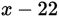• B.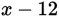• C.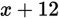• D.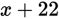Explanation:

B

Difficulty: Easy

Category: Passport to Advanced Math / Exponents

Strategic Advice: When you see a polynomial being divided by another polynomial, don't automatically assume that you need to use long division (unless you just happen to like long division). Factoring may also be an option, especially when one of the polynomials is quadratic.

Getting to the Answer: Write the division as a fraction. Then, factor the numerator to see if anything cancels nicely. To factor the numerator, look for factors of -24 that add up to -10 (which are -12 and +2).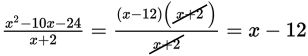The quotient is x - 12, which is (B).